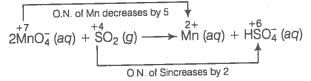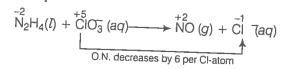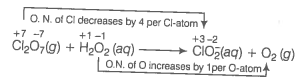22. Calculate the oxidation number of phosphorus in the following species.

(a) ${\mathrm{HPO}}_{3}^{2-}$

(b) ${\mathrm{PO}}_{4}^{3-}$

(a) Ion electron method Write the skeleton equation for the given reaction.
${\mathrm{MnO}}_{4}^{-}\left(\mathrm{aq}\right)+{\mathrm{SO}}_{2}\left(\mathrm{g}\right)\to {\mathrm{Mn}}^{2+}\left(\mathrm{aq}\right)+{\mathrm{HSO}}_{4}^{-}\left(\mathrm{aq}\right)$
Find out the elements which undergo change in O.N.Divide the given skeleton into two half equations.
Reduction half equation : ${\mathrm{MnO}}_{4}^{-}\left(\mathrm{aq}\right)\to {\mathrm{Mn}}^{2+}\left(\mathrm{aq}\right)$
Oxidation half equation : ${\mathrm{SO}}_{2}\left(\mathrm{g}\right)\to {\mathrm{HSO}}_{4}^{-}\left(\mathrm{aq}\right)$
To balance reduction half equation
In acidic medium, balance H and O-atoms
${\mathrm{MnO}}_{4}^{-}\left(\mathrm{aq}\right)+8{\mathrm{H}}^{+}+5{\mathrm{e}}^{-}\to {\mathrm{Mn}}^{2+}\left(\mathrm{aq}\right)+{\mathrm{H}}_{2}\mathrm{O}\left(\mathrm{l}\right)$
To balance the complete reaction
$\frac{2{\mathrm{MnO}}_{4}^{-}\left(\mathrm{aq}\right)+16{\mathrm{H}}^{+}+10{\mathrm{e}}^{-}\to 2{\mathrm{Mn}}^{2+}\left(\mathrm{aq}\right)+8{\mathrm{H}}_{2}\mathrm{O}\left(\mathrm{l}\right)\phantom{\rule{0ex}{0ex}}5{\mathrm{SO}}_{2}\left(\mathrm{g}\right)+10{\mathrm{H}}_{2}\mathrm{O}\left(\mathrm{l}\right)\to 5{\mathrm{HSO}}_{4}^{-}\left(\mathrm{aq}\right)+15{\mathrm{H}}^{+}\left(\mathrm{aq}\right)+10{\mathrm{e}}^{-}}{2{\mathrm{MnO}}_{4}^{-}\left(\mathrm{aq}\right)+5{\mathrm{SO}}_{2}\left(\mathrm{g}\right)+2{\mathrm{H}}_{2}\mathrm{O}\left(\mathrm{l}\right)+{\mathrm{H}}^{+}\left(\mathrm{aq}\right)\to 2{\mathrm{Mn}}^{2+}\left(\mathrm{aq}\right)+5{\mathrm{HSO}}_{4}^{-}\left(\mathrm{aq}\right)}$
(b) Oxidation number method Write the skeleton equation for the given reaction.
${\mathrm{N}}_{2}{\mathrm{H}}_{4}\left(\mathrm{l}\right)+{\mathrm{ClO}}_{3}^{-}\left(\mathrm{aq}\right)\to \mathrm{NO}\left(\mathrm{g}\right)+{\mathrm{Cl}}^{-}\left(\mathrm{g}\right)$
O.N. increases by 4 per N-atomMultiply NO by 2 because in N2H4 there are 2N atoms
${\mathrm{N}}_{2}{\mathrm{H}}_{4}\left(\mathrm{l}\right)+{\mathrm{ClO}}_{3}^{-}\left(\mathrm{aq}\right)\to 2\mathrm{NO}\left(\mathrm{g}\right)+{\mathrm{Cl}}^{-}\left(\mathrm{g}\right)$
Total increase in O.N. of
Total decrease in O.N. of
Therefore, to balance increase or decrease in O.N. multiply ${\mathrm{N}}_{2}{\mathrm{H}}_{4}$ by 3, 2NO by 3 and  by 4
$3{\mathrm{N}}_{2}{\mathrm{H}}_{4}\left(\mathrm{l}\right)+4{\mathrm{ClO}}_{3}^{-}\left(\mathrm{aq}\right)\to 6\mathrm{NO}\left(\mathrm{g}\right)+4{\mathrm{Cl}}^{-}\left(\mathrm{g}\right)$
Balance O and H-atoms by adding $6{\mathrm{H}}_{2}\mathrm{O}$ to RHS
$3{\mathrm{N}}_{2}{\mathrm{H}}_{4}\left(\mathrm{l}\right)+4{\mathrm{ClO}}_{3}^{-}\left(\mathrm{aq}\right)\to 6\mathrm{NO}\left(\mathrm{g}\right)+4{\mathrm{Cl}}^{-}\left(\mathrm{g}\right)+6{\mathrm{H}}_{2}\mathrm{O}\left(\mathrm{l}\right)$
(c) Ion electron method Write the skeleton equation for the given reaction.
${\mathrm{Cl}}_{2}{\mathrm{O}}_{7}\left(\mathrm{g}\right)+{\mathrm{H}}_{2}{\mathrm{O}}_{2}\left(\mathrm{aq}\right)\to {\mathrm{ClO}}_{2}^{-}\left(\mathrm{aq}\right)+{\mathrm{O}}_{2}\left(\mathrm{g}\right)$
Find out the elements which undergo a change in O.N.Divide the given skeleton equation into two half equations.
Reduction half equation : ${\mathrm{Cl}}_{2}{\mathrm{O}}_{7}\to {\mathrm{ClO}}_{2}^{-}$
Oxidation half equation : ${\mathrm{H}}_{2}{\mathrm{O}}_{2}\to {\mathrm{O}}_{2}$
To balance the reduction half equation
${\mathrm{Cl}}_{2}{\mathrm{O}}_{7}\left(\mathrm{g}\right)+6{\mathrm{H}}^{+}\left(\mathrm{aq}\right)+8{\mathrm{e}}^{-}\to 2{\mathrm{ClO}}_{2}^{-}\left(\mathrm{aq}\right)+3{\mathrm{H}}_{2}\mathrm{O}\left(\mathrm{l}\right)$
To balance the oxidation half equation
${\mathrm{H}}_{2}{\mathrm{O}}_{2}\left(\mathrm{aq}\right)\to {\mathrm{O}}_{2}\left(\mathrm{g}\right)+2{\mathrm{H}}^{+}+2{\mathrm{e}}^{-}$
To balance the complete reaction
$\frac{{\mathrm{Cl}}_{2}{\mathrm{O}}_{7}\left(\mathrm{g}\right)+6{\mathrm{H}}^{+}\left(\mathrm{aq}\right)+8{\mathrm{e}}^{-}\to 2{\mathrm{ClO}}_{2}^{-}\left(\mathrm{aq}\right)+3{\mathrm{H}}_{2}\mathrm{O}\left(\mathrm{l}\right)\phantom{\rule{0ex}{0ex}}4{\mathrm{H}}_{2}{\mathrm{O}}_{2}\left(\mathrm{aq}\right)\to 4{\mathrm{O}}_{2}\left(\mathrm{g}\right)+8{\mathrm{H}}^{+}+8{\mathrm{e}}^{-}}{{\mathrm{Cl}}_{2}{\mathrm{O}}_{7}\left(\mathrm{g}\right)+4{\mathrm{H}}_{2}{\mathrm{O}}_{2}\left(\mathrm{aq}\right)\to 2{\mathrm{ClO}}_{2}^{-}\left(\mathrm{aq}\right)+3{\mathrm{H}}_{2}\mathrm{O}\left(\mathrm{l}\right)+4{\mathrm{O}}_{2}\left(\mathrm{g}\right)+2{\mathrm{H}}^{+}+\left(\mathrm{aq}\right)}$
This represents the balanced redox reaction.